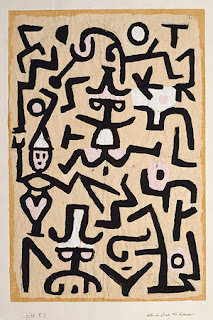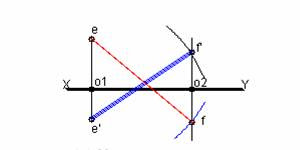Tips for Projections of PointsI quadrant(Point above H.P & in front of V.P)

Front view above XY reference line – Denoted by corresponding small letters with dash(‘)
Distance with respect to H.P

Top view below XY refernce line – Denoted by corresponding small letter only
Distance with respect to V.P

II quadrant (Point above H.P & behind V.P)

Front view above XY reference line – Denoted by corresponding small letters with dash(‘)
Distance with respect to H.P

Top view also above XY refernce line – Denoted by corresponding small letter only
Distance with respect to V.P

III quadrant(Point below H.P & behind V.P)

Front view below XY reference line – Denoted by corresponding small letters with dash(‘)
Distance with respect to H.P

Top view above XY refernce line – Denoted by corresponding small letter only
Distance with respect to V.P

IV quadrant (Point below H.P & in front of V.P)

Front view below XY reference line – Denoted by corresponding small letters with dash(‘)
Distance with respect to H.P

Top view below XY refernce line – Denoted by corresponding small letter only
Distance with respect to V.P

(I thank PSNACET students who developed and circulated the above tips informally)

Example Problem.

Some times combination of two points may be given in the problem like the following one

7.
A point E is 20mm below HP and 30mm behind VP. Another point F is in front of VP and above HP. The distance between the projectors of the points is 60mm. Determine the position of point F, if the lengths of the lines joining the plans and elevations of the points E and F are 80mm and 90mm respectively. (KVN.Ex.No.7, Page.No.?? )As usual the projections of point E namely e and e’ are fixed.

Mark o2 as o1 o2 = 60mm. With e as centre and ef = 80mm as radius cut an arc on a perpendicular to XY line from o2 meeting it below XY. The meeting point f is the plan of the point F.(since this point lies in III quadrant)

Similarly with e’ as centre and radius e’ f’=90mm cut an arc on the perpendicular to XY from o2 meeting it above XY. The meeting point f’ is the elevation of point F.

Answer: Position of the point F

Measure o2 f’ to represent the distance of the point F above HP.

Measure o2 f to represent the distance of point F in front of VP.

1.Tej Shah